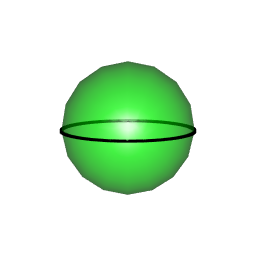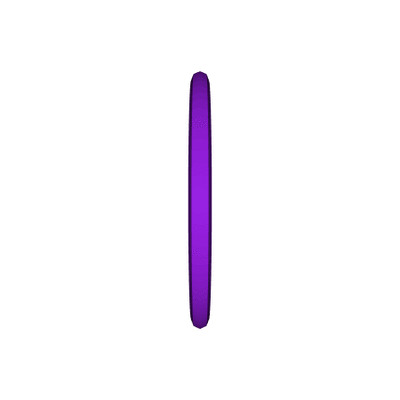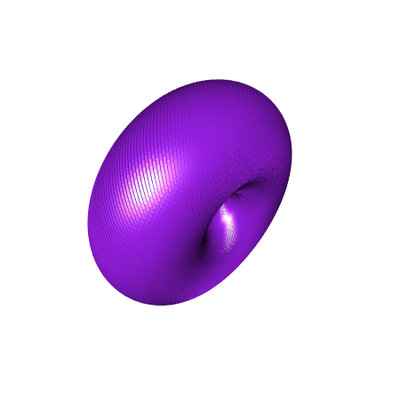# Hopf Torus (1/3): the equatorial case

Posted on May 1, 2018 by Stéphane Laurent
Tags: R, graphics, rgl

In this series of three articles, we will show how to construct Hopf tori.

Hopf tori take their origins in the Hopf map $$S^3 \to S^2$$ defined by $H(p) = \begin{pmatrix} 2(p_2 p_4 - p_1 p_3) \\ 2(p_1 p_4 + p_2 p_3) \\ p_1^2 + p_2^2 - p_3^2 - p_4^2 \\ \end{pmatrix}.$

Obviously, this map is not bijective. Its main property is that it sends each great circle of $$S^3$$ to a same point of $$S^2$$. Thus, it has, say a “pseudo-inverse” $$H^{-1} \colon S^2 \times S^1 \to S^3$$ defined by $H^{-1}(q,t) = \frac{1}{\sqrt{2(1+q_3)}} \begin{pmatrix} q_1 \cos t + q_2 \sin t \\ \sin t (1+q_3) \\ \cos t (1+q_3) \\ q_1 \sin t - q_2 \cos t \\ \end{pmatrix}.$

Now let’s introduce the stereographic projection: $Stereo \colon S^3 -> \mathbb{R}^3 \\ Stereo \, x = \frac{1}{1-x_4}(x_1, x_2, x_3).$

Then, we can draw a circle in $$\mathbb{R}^3$$ as follows:

• take a point $$q$$ on $$S^2$$;

• calculate the great circle on $$S^3$$ by applying $$H^{-1}(q, S^1)$$;

• maps this great circle to $$\mathbb{R}^3$$ with the stereographic projection.

In this article, we will show what happens when we take for $$q$$ a point on the equator on $$S^2$$. Below are the necessary R functions.

hopfinverse <- function(q, t){
1/sqrt(2*(1+q)) * c(q*cos(t)+q*sin(t),
sin(t)*(1+q),
cos(t)*(1+q),
q*sin(t)-q*cos(t))
}
stereog <- function(x){
c(x, x, x)/(1-x)
}

This is the sphere with the equator:Each point of the equator is mapped to a circle, and the circles form a torus:

library(rgl)
view3d(45,45)
t_ <- seq(0, 2*pi, len=200)
phi <- 0
theta_ <- seq(0, 2*pi, len=300)
for(theta in theta_){
circle4d <- sapply(t_, function(t){
hopfinverse(c(cos(theta)*cos(phi), sin(theta)*cos(phi), sin(phi)), t)
})
circle3d <- t(apply(circle4d, 2, stereog))
}